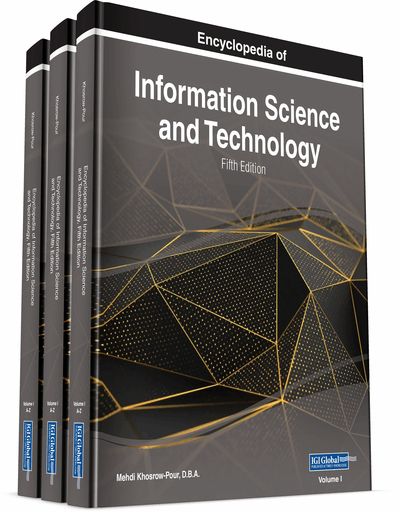# N-Tuple Algebra as a Generalized Theory of Relations

Boris A. Kulik, Alexander Y. Fridman
DOI: 10.4018/978-1-7998-3479-3.ch048
OnDemand:
(Individual Chapters)
Available
\$37.50
No Current Special Offers

## Abstract

In ITs, analysis of heterogeneous information often necessitates unification of presentation forms and processing procedures for such data. To solve this problem, one needs a universal structure, which allows reducing various data and knowledge models to a single mathematical model with unified analysis methods. Such a universal structure is the relation, which is mainly associated with relational algebra. However, relations can model as different, at first glance, mathematical objects as graphs, networks, artificial intelligence structures, predicates, logical formulas, etc. Representation and analysis of such structures and models requires for more expressive means and methods than relational algebra provides. So, with a view to developing a general theory of relations, the authors propose n-tuple algebra (NTA) that allows for formalizing a wide set of logical problems (deductive, abductive, and modified reasoning; modeling uncertainties; and so on). This paper considers matters of metrization and clustering for NTA objects with ordered domains of attributes.
Chapter Preview
Top

## Background

Nowadays, intelligence systems include two types of dissimilar objects, namely databases (DBs) and knowledge bases (KBs). Their structures are fundamentally different, since their construction is based on different theoretical approaches. Modern data structures (numbers, vector graphs, networks, texts, etc.) exploit algebraic techniques (Wirth, 1976). As for KBs, their basic models (predicates, frames, semantic networks, rules) are built on the basis of declarative approaches (Russel & Norvig, 2003). This discrepancy results in significant differences of programming systems for DBs and KBs and, accordingly, in large expenditures of time and money to couple DBs and KBs in one software system. Conventional cases to attempt this integration are mostly accomplished within declarative approaches. An example is the deductive database model (Ullman, 1989; Ceri, Gotlob, & Tanca, 1990), which is based on a modified Prolog programming language intended for computer implementation of the declarative approach.

Conversely, some shortcomings inhere in this approach. One of the main problems is that when using a declarative approach, many tasks of logical analysis need to be reduced to satisfiability checks for a certain logical formula where only two possible answers (“yes” or “no”) are possible. Such a reduction is not simple. Moreover, it is unrealizable in cases when one needs to not only receive a binary answer but also to estimate the value of some parameters in the formal system or to assess the structure and/or number of objects that satisfy the given conditions.

When modeling and analyzing modifiable reasoning (i.e., reasoning with hypotheses and abductive conclusions), many researchers recommend using non-classical logic (default logic, non-monotonic logic, etc.) (Russel & Norvig, 2003), since it is believed that classical logic is ill-suited for solving such problems. However, in many cases, interpretations of non-classical logics are either missing or not corresponding to the semantics of the objects being modeled. There are reasons to assume that the difficulties of modeling modifiable reasoning within the framework of classical logic are not due to the shortcomings of this logic, but to the specifics of the declarative approach.

Algebraic approach to logical modeling can be originated from Aristotle’s syllogistics and Boole’s logical matrices, in more recent times only Arkady Zakrevskij (for instance, see (Zakrevskij & Gavrilov, 1969)) investigated set-theoretical approach to logic and proposed a programming language LYaPAS (Logical Language for Representation of Synthesis Algorithms) oriented toward programming of synthesis algorithms for finite-state and discrete devices. However, this language does not provide any means for logical inference.

## Key Terms in this Chapter

N-Tuple Algebra: An algebraic system whose support is an arbitrary set of n -ary relations expressed by specific structures, namely, C -n-tuple, C -system, D -n-tuple, and D -system. These structures provide a compact expression for sets of elementary n -tuples.

Generalized Operations and Relations: They differ from similar operations and relations of algebra of sets by the only feature: NTA objects (operands) are reduced to the same relation diagram before executing these operations or checking the relations.

C-n-Tuple: An n -tuple of components defined in a certain relation diagram; domain of each component is a subset of the domain of the corresponding attribute.

Collisions: Situations occurring during defeasible reasoning when some new knowledge (hypothesis) is inputted. Such situations can be recognized as violations of some formally expressed rules and/or limitations which control consistency and meaning content of a logical system.

D-n-Tuple: An n -tuple of components enclosed in reversed square brackets and equal to a diagonal C -system with the same diagonal components.

C-System: A set of homotypic C -n-tuples that are denoted as a matrix in square brackets and equal to the intersection of these C -n-tuples. The rows of this matrix are C -n-tuples.

D-System: A set of homotypic D -n-tuples equal to the intersection of these D -n-tuples.

## Complete Chapter List

Search this Book:
Reset